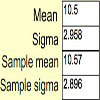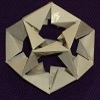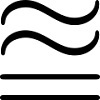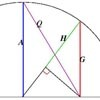# Resources tagged with: Inequalities

Filter by: Content type:
Age range:
Challenge level:

### There are 41 results

Broad Topics > Algebraic expressions, equations and formulae > Inequalities### Random Inequalities

##### Age 16 to 18 Challenge Level:

Can you build a distribution with the maximum theoretical spread?### Shades of Fermat's Last Theorem

##### Age 16 to 18 Challenge Level:

The familiar Pythagorean 3-4-5 triple gives one solution to (x-1)^n + x^n = (x+1)^n so what about other solutions for x an integer and n= 2, 3, 4 or 5?### Unit Interval

##### Age 16 to 18 Short Challenge Level:

Can you prove our inequality holds for all values of x and y between 0 and 1?### Code to Zero

##### Age 16 to 18 Challenge Level:

Find all 3 digit numbers such that by adding the first digit, the square of the second and the cube of the third you get the original number, for example 1 + 3^2 + 5^3 = 135.### Eyes Down

##### Age 16 to 18 Challenge Level:

The symbol [ ] means 'the integer part of'. Can the numbers [2x]; 2[x]; [x + 1/2] + [x - 1/2] ever be equal? Can they ever take three different values?### ' Tis Whole

##### Age 14 to 18 Challenge Level:

Take a few whole numbers away from a triangle number. If you know the mean of the remaining numbers can you find the triangle number and which numbers were removed?### Inner Equality

##### Age 16 to 18 Short Challenge Level:

Can you solve this inequalities challenge?### Reciprocals

##### Age 16 to 18 Challenge Level:

Prove that the product of the sum of n positive numbers with the sum of their reciprocals is not less than n^2.### Diverging

##### Age 16 to 18 Challenge Level:

Show that for natural numbers x and y if x/y > 1 then x/y>(x+1)/(y+1}>1. Hence prove that the product for i=1 to n of [(2i)/(2i-1)] tends to infinity as n tends to infinity.### Big, Bigger, Biggest

##### Age 16 to 18 Challenge Level:

Which is the biggest and which the smallest of $2000^{2002}, 2001^{2001} \text{and } 2002^{2000}$?##### Age 16 to 18 Challenge Level:

Can you find a quadratic equation which passes close to these points?### Farey Neighbours

##### Age 16 to 18 Challenge Level:

Farey sequences are lists of fractions in ascending order of magnitude. Can you prove that in every Farey sequence there is a special relationship between Farey neighbours?### Exhaustion

##### Age 16 to 18 Challenge Level:

Find the positive integer solutions of the equation (1+1/a)(1+1/b)(1+1/c) = 2### Thousand Words

##### Age 16 to 18 Challenge Level:

Here the diagram says it all. Can you find the diagram?### Unit Interval

##### Age 14 to 18 Challenge Level:

Take any two numbers between 0 and 1. Prove that the sum of the numbers is always less than one plus their product?### Proofs with Pictures

##### Age 14 to 18

Some diagrammatic 'proofs' of algebraic identities and inequalities.### Giants

##### Age 16 to 18 Challenge Level:

Which is the bigger, 9^10 or 10^9 ? Which is the bigger, 99^100 or 100^99 ?### Tetra Inequalities

##### Age 16 to 18 Challenge Level:

Prove that in every tetrahedron there is a vertex such that the three edges meeting there have lengths which could be the sides of a triangle.### In Between

##### Age 16 to 18 Challenge Level:

Can you find the solution to this algebraic inequality?##### Age 16 to 18 Challenge Level:

Find all positive integers a and b for which the two equations: x^2-ax+b = 0 and x^2-bx+a = 0 both have positive integer solutions.### Power Up

##### Age 16 to 18 Challenge Level:

Show without recourse to any calculating aid that 7^{1/2} + 7^{1/3} + 7^{1/4} < 7 and 4^{1/2} + 4^{1/3} + 4^{1/4} > 4 . Sketch the graph of f(x) = x^{1/2} + x^{1/3} + x^{1/4} -x### Squareness

##### Age 16 to 18 Challenge Level:

The family of graphs of x^n + y^n =1 (for even n) includes the circle. Why do the graphs look more and more square as n increases?### Comparing Continued Fractions

##### Age 16 to 18 Challenge Level:

Which of these continued fractions is bigger and why?### Integral Inequality

##### Age 16 to 18 Challenge Level:

An inequality involving integrals of squares of functions.### Rationals Between...

##### Age 14 to 16 Challenge Level:

What fractions can you find between the square roots of 65 and 67?### Without Calculus

##### Age 16 to 18 Challenge Level:

Given that u>0 and v>0 find the smallest possible value of 1/u + 1/v given that u + v = 5 by different methods.### Not Continued Fractions

##### Age 14 to 18 Challenge Level:

Which rational numbers cannot be written in the form x + 1/(y + 1/z) where x, y and z are integers?### Two Cubes

##### Age 14 to 16 Challenge Level:

Two cubes, each with integral side lengths, have a combined volume equal to the total of the lengths of their edges. How big are the cubes? [If you find a result by 'trial and error' you'll need to. . . .### After Thought

##### Age 16 to 18 Challenge Level:

Which is larger cos(sin x) or sin(cos x) ? Does this depend on x ?### Integral Sandwich

##### Age 16 to 18 Challenge Level:

Generalise this inequality involving integrals.### Climbing

##### Age 16 to 18 Challenge Level:

Sketch the graphs of y = sin x and y = tan x and some straight lines. Prove some inequalities.### Biggest Enclosure

##### Age 14 to 16 Challenge Level:

Three fences of different lengths form three sides of an enclosure. What arrangement maximises the area?### Square Mean

##### Age 14 to 16 Challenge Level:

Is the mean of the squares of two numbers greater than, or less than, the square of their means?### All-variables Sudoku

##### Age 11 to 18 Challenge Level:

The challenge is to find the values of the variables if you are to solve this Sudoku.### Almost Total Inequality

##### Age 14 to 16 Challenge Level:### Classical Means

##### Age 16 to 18 Challenge Level:

Use the diagram to investigate the classical Pythagorean means.##### Age 14 to 16 Challenge Level:

Kyle and his teacher disagree about his test score - who is right?### Fracmax

##### Age 14 to 16 Challenge Level:

Find the maximum value of 1/p + 1/q + 1/r where this sum is less than 1 and p, q, and r are positive integers.### Approximating Pi

##### Age 14 to 18 Challenge Level:

By inscribing a circle in a square and then a square in a circle find an approximation to pi. By using a hexagon, can you improve on the approximation?### Discrete Trends

##### Age 16 to 18 Challenge Level:

Find the maximum value of n to the power 1/n and prove that it is a maximum.### Tet-trouble

##### Age 14 to 16 Challenge Level:

Is it possible to have a tetrahedron whose six edges have lengths 10, 20, 30, 40, 50 and 60 units?